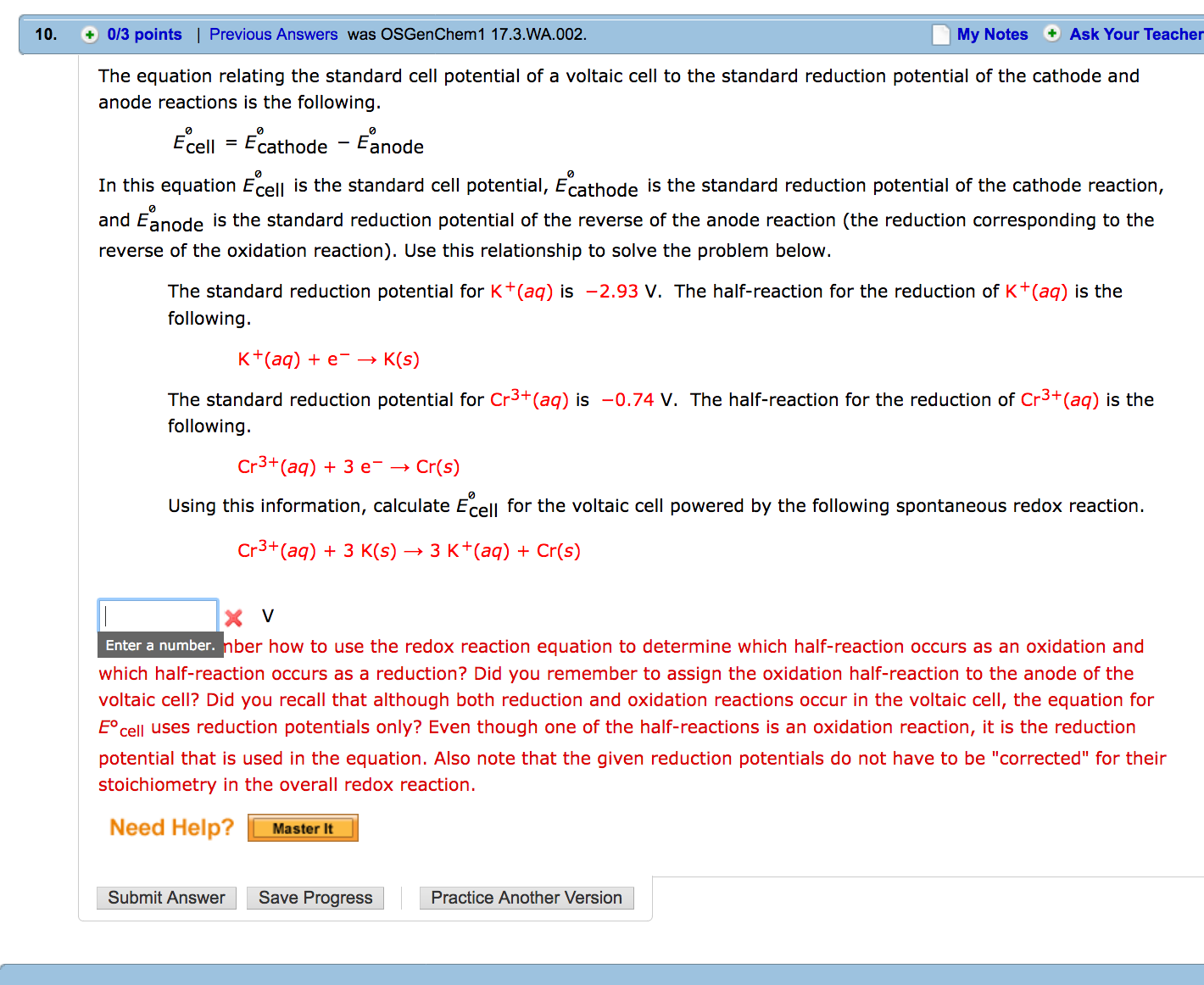# The equation relating the standard cell potential of a voltaic cell to the standard reduction potential of the cathode and anode reactions is the following. E0cell = Eocathode - Eoanode In this equation Eocell is the standard cell potential, Eocathode is the standard reduction potential of the cathode reaction, and Eanode is the standard reduction potential of the reverse of the anode reaction (the reduction corresponding to the reverse of the oxidation reaction). Use this relationship to solve the problem below.